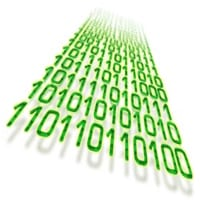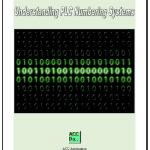# What Everybody Ought to Know About PLC (Programmable Logic Controller) Numbering Systems

Programmable Logic Controllers (PLC) are the same as computers. They only understand two conditions; on and off. (1 or 0 / Hi or Low/ etc.) This is known as binary. The PLC will only understand binary but we need to display, understand and use other numbering systems to make things work. Let’s look at the following common numbering systems.

Binary has a base of two (2). Base means the number of symbols used. In binary the symbols are 1 or 0. Each binary symbol can be referred to as a bit. Putting multiple bits together will give you something that looks like this: 100101112. The 2 represents the number of symbols/binary notation. Locations of the bits will indicate weight of the number. The weight of the number is just the number to the power of the position. Positions always start at 0. The right hand bit is the ‘least significant bit’ and the left hand bit is the ‘most significant bit’.Let’s look back at our example to determine what the value of the binary number is:
100101112 =
We start with the least significant bit and work our way to the most significant bit.
1 x 2= 1 x 1 = 1
1 x 2= 1 x 2 = 2

1 x 2= 1 x 2 x 2 = 4
0 x 2= 0 x 2 x 2 x 2 = 0
1 x 2= 1 x 2 x 2 x 2 x 2 = 16
0 x 2= 0 x 2 x 2 x 2 x 2 x 2 = 0
0 x 2= 0 x 2 x 2 x 2 x 2 x 2 x 2 = 0
1 x 2= 1 x 2 x 2 x 2 x 2 x 2 x 2 x 2 = 128
100101112  = 1 + 2  + 4 + 16 + 128
100101112  = 151
Note that the we just converted the binary number to our decimal numbering system. The decimal numbering system is not written with a base value of 10 because this is universally understood.
To be sure we have the concept down, let’s take a look at our decimal numbering system the same way as we did the binary.
Decimal has a base of ten (10). The symbols are 0, 1, 2, 3, 4, 5, 6, 7, 8 and 9.
15110 =
1 x 10= 1 x 1 = 1

5 x 10= 5 x 10 = 50
1 x 10= 1 x 10 x 10 = 100
15110 = 1 + 50 + 100
151 = 151

Hexadecimal has a base of sixteen (16). The symbols are  0, 1, 2, 3, 4, 5, 6, 7, 8, 9, A, B, C, D, E and F. Hexadecimal is used to represent binary numbers. F16 = 1111
Every for bits of binary represent one hexadecimal digit.
In our original binary number we now can convert this to hexadecimal.
100101112
The least significant four bits are:
01112 =
1 x 2= 1 x 1 = 1
1 x 2= 1 x 2 = 2

1 x 2= 1 x 2 x 2 = 4

0 x 2= 0 x 2 x 2 x 2 = 0

0111= 1 + 2 + 4 + 0 = 716
The most significant four bits are:
1001=
1 x 2= 1 x 1 = 1
0 x 2= 0 x 2 = 0
0 x 2= 0 x 2 x 2 = 0

1 x 2= 1 x 2 x 2 x 2 = 8

1001= 1 + 0 + 0 + 8 = 916
Therefore:
100101112 = 9716
We can now convert this hexadecimal number back into decimal
9716 =

7 x 16= 7 x 1 = 7
9 x 16= 9 x 16 = 144
9716 = 7 + 144 = 151

The following chart will show all of the combinations for 4 bits (nibble) of binary. Its shows the Binary, Decimal and Hexadecimal (Hex) values. It is interesting to not that Hex is used because you still have only one digit (Place Holder) to represent the nibble of information.

 Binary Decimal Hexadecimal Binary Decimal Hexadecimal 0000 00 0 1000 08 8 0001 01 1 1001 09 9 0010 02 2 1010 10 A 0011 03 3 1011 11 B 0100 04 4 1100 12 C 0101 05 5 1101 13 D 0110 06 6 1110 14 E 0111 07 7 1111 15 F
ASCII (American Standard Code for Information Interchange)
Two nibbles (8 bits of data) together form a byte. A byte is what computers (PLC) use to store and use individual information. So it will take one unique byte to represent each individual numbers, letters (upper and lower case), punctuation etc. www.AsciiTable.com
Example:
Chr ‘A’ = 4116 = 010000012
Chr ‘a’ = 6116 = 011000012
Chr ‘5’ = 3516 = 001101012
Each time you hit a key on your keyboard, the following 8 bits of data get sent.
A word is made up of two bytes, or 4 nibbles, or 16 bits of data. Words are used in the PLC for holding information. The word can also be referred to as an integer.
Long word / Double word is made up of 4 bytes, or 8 nibbles, or 32 bits of data. Long words are used for instructions in the PLC like math.
So far we have talked about unsigned words. (Positive numbers)
Signed words can hold negative numbers. Bit 15 (most significant bit) of a word is used to determine if the word is negative or not.
The following table shows you the signed vs unsigned numbers that can be represented in the PLC.
 HEX 8000 BFFF FFFE FFFF 0000 3FFF 7FFE 7FFF Signed -32768 -16385 -0002 -0001 00000 16383 32766 32767 Unsigned 32768 49151 65534 65535 00000 16383 32766 32767
Memory retentiveness:
When working with PLC’s look at the memory tables to determine what will happen if power is removed from the device. Will the bits go all off or retain their prior state?
Usually there will be areas that can be used in the PLC for both conditions.
As you can see PLC numbering systems and computers are very much related and it all boils down to individual bits turning on and off. The interpretation of these bits will determine what the value will be.

Reference:
Let me know your thoughts, or questions that you have on PLC numbering systems.

Thank you,
Garry

If you’re like most of my readers, you’re committed to learning about technology. Numbering systems used in PLC’s are not difficult to learn and understand. We will walk through the numbering systems used in PLCs. This includes Bits, Decimal, Hexadecimal, ASCII and Floating Point.

To get this free article, subscribe to my free email newsletter.

Use the information to inform other people how numbering systems work. Sign up now.## 2 thoughts on “What Everybody Ought to Know About PLC (Programmable Logic Controller) Numbering Systems”

1.Supriadi says:

do you mind if we can download the plc software that is discussed so that we can immediately understand it and run it thanks

1.garrys says: